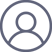高宏2023-2-5 20:38X(t)=a是《随机过程》教科书定义首中时Ta的前提条件，对X(t)=a两边求取数学期望，有

E[X(t)]=E[a]

E[X(t)]=0

E[a]=a

0=a